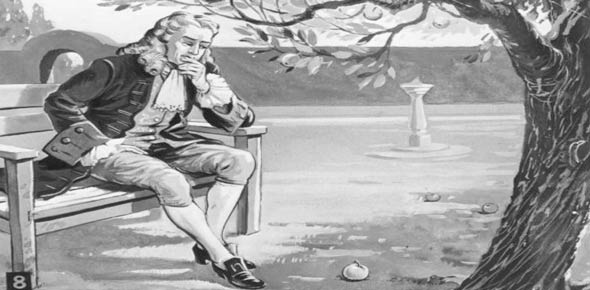# Physics : Motion, Newton Laws

3 Questions | Total Attempts: 60SettingsThis test is on physics grade 7 good luck

Related Topics
• 1.
State newton's second law.
• A.

Acceleration of an object increase with increased force and decreases with decreased motion.

• B.

Acceleration of an object increase with increased force and decreases with decreased acceleration.

• C.

Acceleration of an object increase with increased force and decreases with decreased force.

• D.

Acceleration of an object increase with increased force and decreases with decreased mass.

• E.

Acceleration of an object increase with increased force and decreases with decreased velocity.

• 2.
What is centripetal force?
• 3.
Newton's third law of motion states that for every action there is equal and opposite           .